# How to Remove the Legend in Matplotlib?

• Last Updated : 01 Feb, 2021

Matplotlib is one of the most popular data visualization libraries present in Python. Using this matplotlib library, if we want to visualize more than a single variable, we might want to explain what each variable represents. For this purpose, there is a function called legend() present in matplotlib library. This legend is a small area on the graph describing what each variable represents.

In order to remove the legend, there are four ways. They are :

• Using .remove()
• Using .set_visible()
• Fix legend_ attribute of the required Axes object = None
• Using label=_nolegend_

Method 1: Using .remove()

Example 1: By using ax.get_legend().remove() method, legend can be removed from figure in matplotlib.

## Python3

 `import` `numpy as np``import` `matplotlib.pyplot as plt` `x ``=` `np.linspace(``-``3``, ``3``, ``100``)``y1 ``=` `np.power(x, ``2``)``y2 ``=` `np.power(x, ``3``)` `fig, ax ``=` `plt.subplots()` `ax.plot(x, y1, c ``=` `'r'``,label ``=` `'x^2'``)``ax.plot(x, y2, c ``=` `'g'``,label ``=` `'x^3'``)` `leg ``=` `plt.legend()` `ax.get_legend().remove()` `plt.show()`

Output :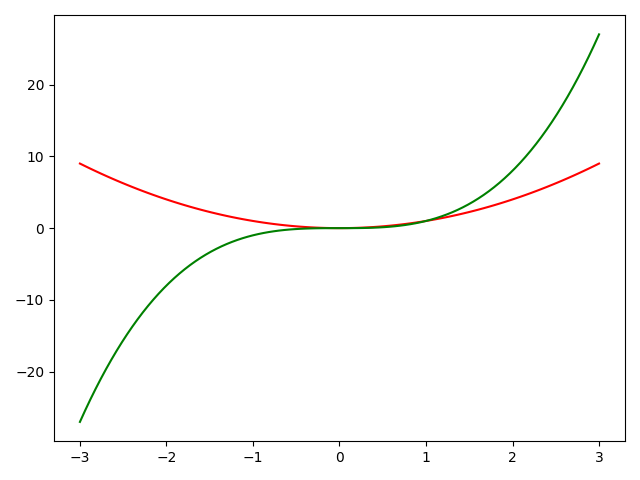We can see that there is no legend in the above figure.

Example 2: More than one subplots :

In the case of more than one subplot, we can mention the required subplot object for which we want to remove the legend. Here, we have written axs.get_legend().remove() which means we are removing legend for second subplot specifically.

## Python3

 `import` `numpy as np``import` `matplotlib.pyplot as plt` `x ``=` `np.linspace(``-``3``, ``3``, ``100``)``y1 ``=` `np.power(x, ``2``)``y2 ``=` `np.power(x, ``3``)` `fig, axs ``=` `plt.subplots(``2``, ``1``)` `axs[``0``].plot(x, y1, c ``=` `'r'``,label ``=` `'x^2'``)``axs[``1``].plot(x, y2, c ``=` `'g'``,label ``=` `'x^3'``)` `axs[``0``].legend(loc ``=` `'upper left'``)``axs[``1``].legend(loc ``=` `'upper left'``)` `axs[``1``].get_legend().remove()`  `plt.show()`

Output :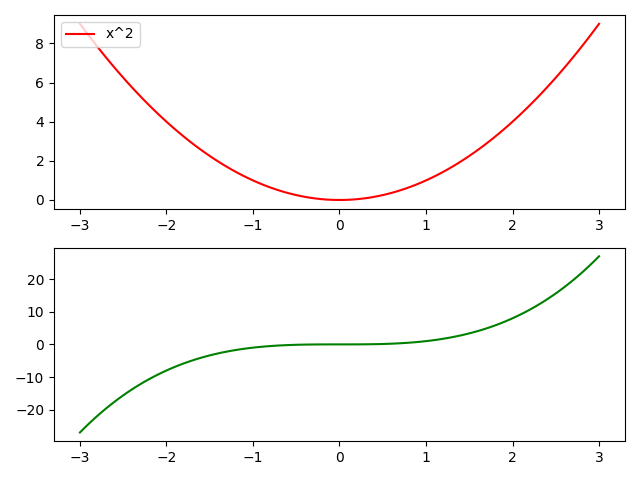In the above figure, we removed the legend for the second subplot specifically. The first subplot will still have a legend.

Method 2: Using set_visible()

Example 1: By using ax.get_legend().set_visible(False) method, legend can be removed from figure in matplotlib.

## Python3

 `import` `numpy as np``import` `matplotlib.pyplot as plt` `x ``=` `np.linspace(``-``3``, ``3``, ``1000``)``y1 ``=` `np.sin(x)``y2 ``=` `np.cos(x)` `fig, ax ``=` `plt.subplots()` `ax.plot(x, y1,c ``=` `'r'``,label ``=` `'Sine'``)``ax.plot(x, y2,c ``=` `'g'``,label ``=` `'Cosine'``)` `leg ``=` `plt.legend()` `ax.get_legend().set_visible(``False``)` `plt.show()`

Output :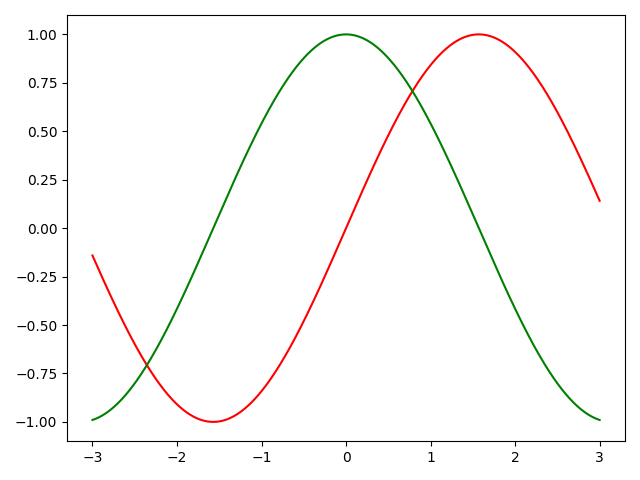We can see that there is no legend in the above figure.

Example-2. More than one subplots  :

In case of more than one subplot, we can mention the required subplot object for which we want to remove the legend. Here, we have written axs.get_legend().set_visible(False) which means we are removing legend for second subplot specifically.

## Python3

 `import` `numpy as np``import` `matplotlib.pyplot as plt` `x ``=` `np.linspace(``-``3``,``3``,``1000``)``y1 ``=` `np.sin(x)``y2 ``=` `np.cos(x)` `fig, axs ``=` `plt.subplots(``2``,``1``)` `axs[``0``].plot(x,y1,c``=``'r'``,label ``=` `'Sine'``)``axs[``1``].plot(x,y2,c``=``'g'``,label ``=` `'Cosine'``)` `axs[``0``].legend(loc``=``'upper left'``)``axs[``1``].legend(loc``=``'upper left'``)` `axs[``1``].get_legend().set_visible(``False``)`  `plt.show()`

Output :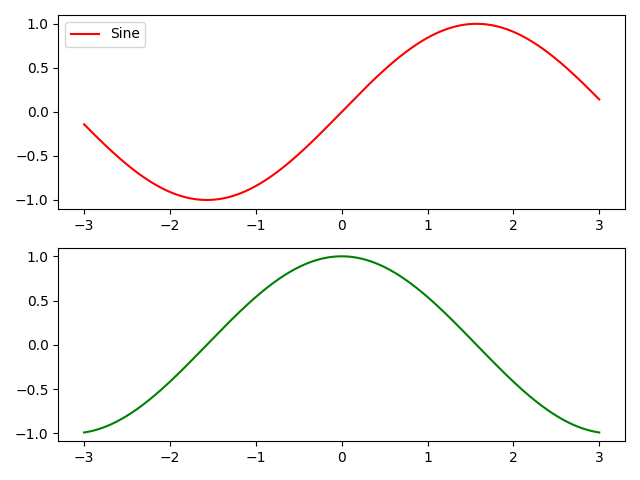In the above figure, we removed legend for the second subplot specifically. The first subplot will still have legend.

Method 3: Fix legend_ attribute of the required Axes object = None :

Example 1: By using ax.legend_ = None, legend can be removed from figure in matplotlib.

## Python3

 `import` `numpy as np``import` `matplotlib.pyplot as plt` `x ``=` `np.linspace(``-``3``, ``3``, ``1000``)``y1 ``=` `np.sin(x)``y2 ``=` `np.cos(x)` `fig, ax ``=` `plt.subplots()` `ax.plot(x, y1,c ``=` `'r'``,label ``=` `'Sine'``)``ax.plot(x, y2,c ``=` `'g'``,label ``=` `'Cosine'``)``leg ``=` `plt.legend()``ax.legend_ ``=` `None` `plt.show()`

Output:We can see that there is no legend in the above figure.

Example 2: More than one subplot:

In the case of more than one subplot, we can mention the required subplot object for which we want to remove the legend. Here, we have written axs.legend_ = None which means we are removing legend for the first subplot specifically.the

## Python3

 `import` `numpy as np``import` `matplotlib.pyplot as plt` `x ``=` `np.linspace(``-``3``, ``3``, ``1000``)``y1 ``=` `np.sin(x)``y2 ``=` `np.cos(x)` `fig, axs ``=` `plt.subplots(``2``, ``1``)` `axs[``0``].plot(x, y1, c ``=` `'r'``,label ``=` `'Sine'``)``axs[``1``].plot(x, y2,c ``=` `'g'``,label ``=` `'Cosine'``)``axs[``0``].legend(loc ``=` `'upper left'``)``axs[``1``].legend(loc ``=` `'upper left'``)``axs[``0``].legend_ ``=` `None` `plt.show()`

Output: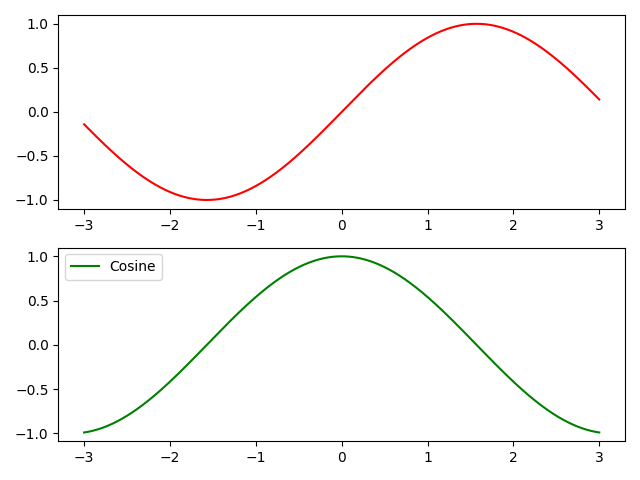In the above figure, we removed legend for the first subplot specifically. The second subplot will still have legend.

Method 4: Using label = _legend_

Example 1: By sending label = ‘_nolegend_’ argument in ax.plot(), legend can be removed from figure in matplotlib.

## Python3

 `import` `numpy as np``import` `matplotlib.pyplot as plt` `x ``=` `np.linspace(``-``3``, ``3``, ``100``)``y1 ``=` `np.power(x, ``2``)``y2 ``=` `np.power(x, ``3``)` `fig, ax ``=` `plt.subplots()` `ax.plot(x, y1, c ``=` `'r'``,label ``=` `'_nolegend_'``)``ax.plot(x, y2,c ``=` `'g'``,label ``=` `'_nolegend_'``)` `leg ``=` `plt.legend()` `plt.show()`

Output: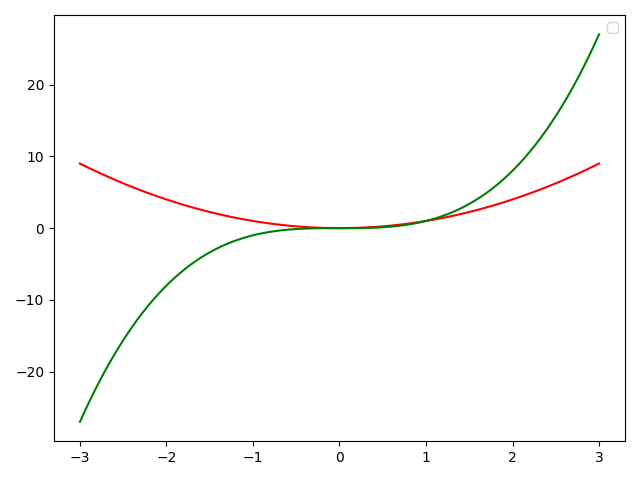Example-2. More than one subplots  :

In case of more than one subplot, we can mention the required subplot object for which we want to remove the legend. Here, we have written axs.plot(x,y1,c=’r’,label = ‘_nolegend_’) which means we are removing legend for first subplot specifically.

## Python3

 `import` `numpy as np``import` `matplotlib.pyplot as plt` `x ``=` `np.linspace(``-``3``,``3``,``100``)``y1 ``=` `np.power(x,``2``)``y2 ``=` `np.power(x,``3``)` `fig, axs ``=` `plt.subplots(``2``,``1``)` `axs[``0``].plot(x,y1,c``=``'r'``,label ``=` `'_nolegend_'``)``axs[``1``].plot(x,y2,c``=``'g'``,label ``=` `'x^3'``)` `axs[``0``].legend(loc``=``'upper left'``)``axs[``1``].legend(loc``=``'upper left'``)`  `plt.show()`

Output :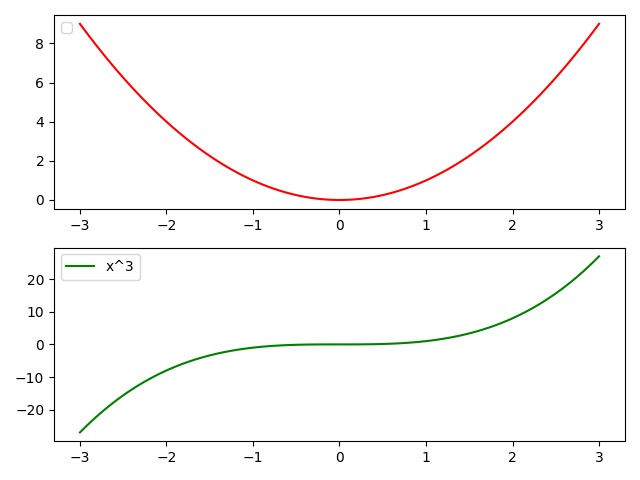In the above figure, we removed legend for the first subplot specifically. The second subplot will still have legend.

My Personal Notes arrow_drop_up